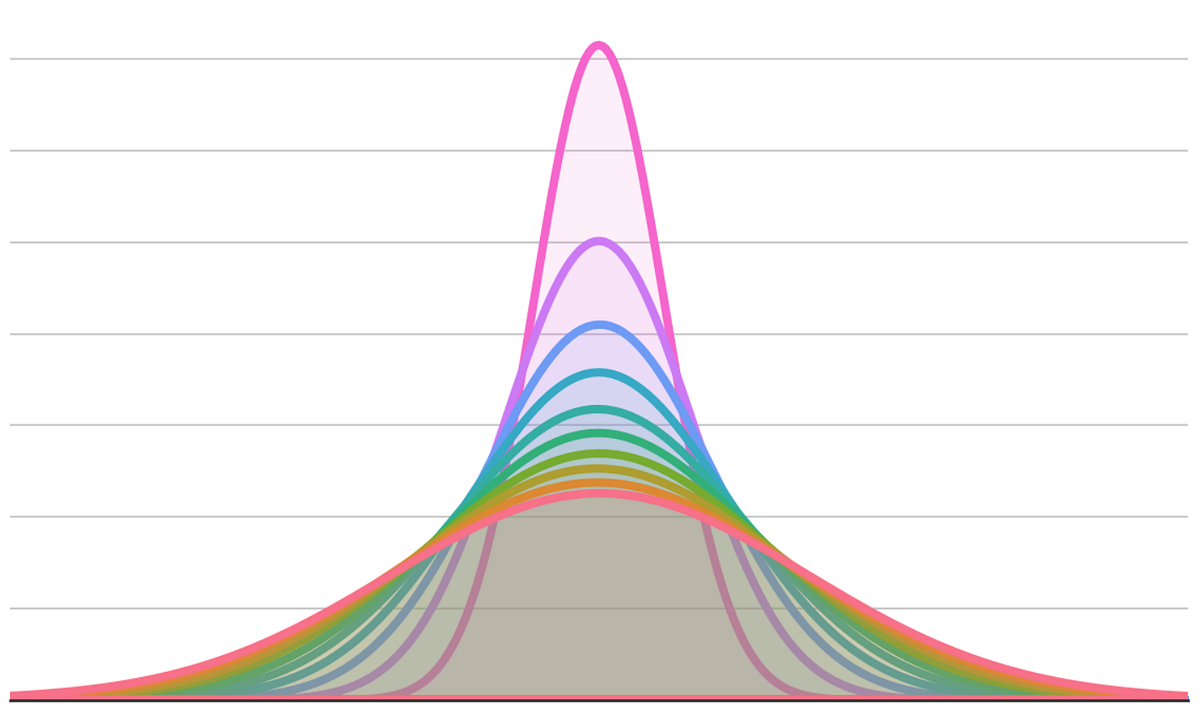# Understanding CUPED §• Author: Matteo Courthoud
• Full Title: Understanding CUPED
• Document Note: CUPED (Controlled-Experiment using Pre-Experiment Data) is a technique used to increase the power of randomized controlled trials in A/B tests, which is essentially a residualized outcome regression. It can be computed via a difference in means or an equivalent formulation. It controls for individual-level variation that is persistent over time and is related to, but not equivalent to Difference-in-Differences and autoregression. Simulations show that CUPED, Difference-in-Differences, and autoregression have similar standard deviations, while the simple difference estimator has a larger standard deviation. CUPED (Controlled-Experiment using Pre-Experiment Data) is a technique used to increase the power of randomized controlled trials in A/B tests. It is essentially a residualized outcome regression and can be computed by regressing the post-treatment outcome on the treatment indicator, or by regressing the pre-treatment outcome and computing the residuals. It is closely related to autoregression and difference-in-differences, but is not equivalent, except in special cases. When randomization is imperfect, difference-in-differences is more efficient than the other methods.
• URL: understanding-cuped-a822523641af

## Highlights §

• CUPED (Controlled-Experiment using Pre-Experiment Data), a technique to increase the power of randomized controlled trials in A/B tests. (View Highlight)
• noticed a similarity with some causal inference methods I was familiar with, such as Difference-in-Differences or regression with control variables. (View Highlight)
• CUPED is essentially a residualized outcome regression (View Highlight)
• We randomly split a set of users into a treatment and control group and we show the ad campaign to the treatment group. Differently from the standard A/B test setting, assume we observe users also before the test. (View Highlight)
• This estimator is unbiased, which means it delivers the correct estimate, on average. However, it can still be improved: we could decrease its variance. Decreasing the variance of an estimator is extremely important since it allows us to • detect smaller effects • detect the same effect, but with a smaller sample size (View Highlight)
• The idea of CUPED is the following. Suppose you are running an AB test and Y is the outcome of interest (revenue in our example) and the binary variable D indicates whether a single individual has been treated or not (ad_campaign in our example). Suppose you have access to another random variable X which is not affected by the treatment and has known expectation 𝔼[X]. (View Highlight)
• the higher the correlation between Y and X, the higher the variance reduction of CUPED. (View Highlight)
• What is the optimal choice for the control variable X? We know that X should have the following properties: • not affected by the treatment • as correlated with Y₁ as possible The authors of the paper suggest using the pre-treatment outcome Y₀ since it gives the most variance reduction in practice. (View Highlight)
• we can compute the CUPED estimate of the average treatment effect as follows:
1. Regress Y₁ on Y₀ and estimate θ̂
2. Compute Ŷ₁ᶜᵘᵖᵉᵈ = Y̅₁ − θ̂ Y̅₀
3. Compute the difference of Ŷ₁ᶜᵘᵖᵉᵈ between treatment and control group (View Highlight)
• The main advantage of diff-in-diff is that it allows to estimate the average treatment effect when randomization is not perfect and the treatment and control group are not comparable. The key assumption is that the difference between the treatment and control groups is constant over time. By taking a double difference, we cancel it out. (View Highlight)
• The most common way to compute the diff-in-diffs estimator is to first reshape the data in a long format or panel format (one observation is an individual i at time period t) and then regress the outcome Y on the full interaction between the post-treatment dummy 𝕀(t=1) and the treatment dummy D. (View Highlight)
• With imperfect treatment assignment, both difference-in-differences and autoregression are unbiased for the true treatment effect, however, diff-in-diffs is more efficient. Both CUPED and simple difference are biased instead. (View Highlight)